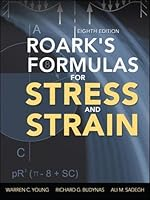# Roark’s Formulas for Stress and Strain, 8th Edition## Book Description

#### THE MOST COMPLETE, UP-TO-DATE GUIDE TO STRESS AND STRAIN FORMULAS

Fully revised throughout, Roark's Formulas for Stress and Strain, Eighth Edition, provides accurate and thorough tabulated formulations that can be applied to the stress of a comprehensive range of structural components. All equations and diagrams of structural properties are presented in an easy-to-use, thumb, through format.

This extensively updated edition contains new chapters on fatigue and fracture mechanics, stresses in fasteners and joints, composite materials, and biomechanics. Several chapters have been expanded and new topics have been added. Each chapter now concludes with a summary of tables and formulas for ease of reference. This is the definitive resource for , engineers, and analysts who need to calculate stress and strain .

ROARK'S FORMULAS FOR STRESS AND STRAIN, EIGHTH EDITION, COVERS:

• of bodies under stress
• and analytical methods
• Numerical and experimental methods
• Tension, compression, shear, and combined stress
• Beams; flexure of straight bars
• Bending of curved beams
• Torsion
• Flat plates
• Columns and other compression members
• Shells of revolution; pressure vessels; pipes
• Bodies in contact undergoing direct bearing and shear stress
• Elastic stability
• and temperature stresses
• Stress concentration factors
• Fatigue and fracture mechanics
• Stresses in fasteners and joints
• Composite materials
• Biomechanics

Chapter 1 Introduction
Chapter 2 Stress And Strain: Important Relationships
Chapter 3 The Behavior Of Bodies Under Stress
Chapter 4 Principles And Analytical Methods
Chapter 5 Numerical Methods
Chapter 6 Experimental Methods
Chapter 7 Tension, Compression, Shear, And Combined Stress
Chapter 8 Beams; Flexure Of Straight Bars
Chapter 9 Curved Beams
Chapter 10 Torsion
Chapter 11 Flat Plates
Chapter 12 Columns And Other Compression Members
Chapter 13 Shells Of Revolution; Pressure Vessels; Pipes
Chapter 14 Bodies Under Direct Bearing And Shear Stress
Chapter 15 Elastic Stability
Chapter 16 Dynamic And Temperature Stresses
Chapter 17 Stress Concentration
Chapter 18 Fatigue And Fracture
Chapter 19 Stresses In Fasteners And Joints
Chapter 20 Composite Materials
Chapter 21 Solid Biomechanics
Appendix A: Properties Of A Plane Area
Appendix B: Mathematical Formulas And Matrices
Appendix C: Glossary